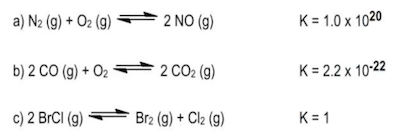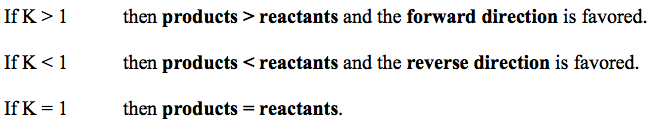Ch.7 Energy, Rate and EquilibriumWorksheetSee all chapters

# The Equilibrium Constant

See all sections
Sections
Nature of Energy
First Law of Thermodynamics
Endothermic & Exothermic Reactions
Bond Energy
Thermochemical Equations
Heat Capacity
Thermal Equilibrium (Simplified)
Hess's Law
Rate of Reaction
Energy Diagrams
Chemical Equilibrium
The Equilibrium Constant
Le Chatelier's Principle
Solubility Product Constant (Ksp)
Spontaneous Reaction
Entropy (Simplified)
Gibbs Free Energy (Simplified)

Equilibrium Constant K is associated with any reaction at equilibrium. Its numerical value determines if reactants or products are more greatly favored within a reaction.

###### The Equilibrium Constant K

Concept #1: Equilibrium Constant

###### Expert Q&A

Ask unlimited questions and get expert help right away.

Transcript

Hey guys! In this brand new video, we're going to take a look at the Equilibrium Constant.
Now we're going to say that the variable we use to illustrate our equilibrium constant is the variable K. And we're going to say K is a number equal to the ratio of products to reactants at a given temperature. Why do I say at a given temperature? Because we're going to say that temperature directly affects our equilibrium constant K. Increasing the temperature will increase our K value, decreasing our temperature will decrease our K value. Now we're going to say it's a ratio of products to reactants so we're going to say that here K = products/reactants. Now we're going to say that K is important because its magnitude tells us how far to the left or to the right our chemical reaction is at a given temperature. So we're going to say if K is greater than 1 then products are favored over reactants. And think about it, this makes sense because we said K = products/reactants so let's say our products are 10 and our reactants are 1, K = 10 definitely greater than 1. We're going to say when products are favored over our reactants that mean we're making more products. How do we make more products? By going in the forward direction. So the forward direction would be favored. Now in the opposite way, if K is less than 1, so let's say we have in this case products are 1 but our reactants are 10, we'd have a K less than 1. So in this case, if K is less than 1 then reactants are favored over products. Which means our reaction is heading in the reverse direction. So the reverse direction would be favored. So just remember these 2 differences, when K is greater than 1, when K is less than 1. Now what if we say K was equal to 1? We know that this is products/reactants so that means our products and reactants would have to be equal, let's say they're both 10. 10/10 is 1. So when K = 1 we're going to say both our reactant and our product amounts are equal to one another. Now we're going to say the equilibrium constant K takes into account all the stats of matter except 2, it doesn't look at solids and it doesn't look at liquids, it ignores those 2 states for matter.

The Equilibrium Constant K is a ratio of products to reactants. It only deals with gaseous or aqueous compounds.Example #1: Write the equilibrium expression for the following reaction:

###### Expert Q&A

Ask unlimited questions and get expert help right away.

Transcript

So it looks at it only cares about really gases and also aqueous. Aqueous just means that the solvent is water, so a compound is dissolved in water. It would be aqueous.
Now knowing this, let's take a look at the following Example. It says:
Write the equilibrium expression for the following reaction.
Here we have 2 and 2 N2O5 (aq) gives us 4 NO2 (aq) + O2 (aq). It's important to look at the phases because remember if it's a solid or a liquid we ignore it. Now we're going to say here K = products/reactants. So we're going to have our products as NO2. And remember you have to use the coefficients in these calculations. The number in front of NO2 is a 4, so that 4 will become the power, so it's going to be (NO2)4 * O2, O2. just has a coefficient of 1 in front of it which we don't have to show, divide it by N2O5, again the coefficient in front of N2O5 is 2 so that becomes a power.

K = products = (NO2)4 * (O2)
reactants (N2O5)2

So we would say that this represents our equilibrium expression or our equilibrium equation. Same thing, equilibrium expression, equilibrium equation.
Now that we've seen this one, let's look at B. For B we're going to say here, we're going to ignore this compound because it's a solid and we're going to ignore this compound because it's a solid. Here we're going to say K = products/, like before. But here's the thing, we're going to say we don't have any products available but you have to put something on top. We're going to say it's equal to 1. Solids and liquids are ignored and in place of them we're going to put 1. We're going to say this because things such as pressured don't really affect solids and liquids like they do gases and aqueous compounds, that's why they're equal to 1 because their number’s being held constant. So we're going to replace solids and liquids with the number 1. And on the bottom we'd have O2.

K = products = 1
reactants (O2)1

Now different way your professor could give you this is, we know there's a 1 here so this could also be or O2 inverse 1. So be aware of both type of answers. Both are correct, both are saying the same thing. Inverse 1 just means 1 over whatever it is.

K = products = 1 or O2-1
reactants (O2)1

For the next one, again we ignore solids. The only thing that we don't ignore is this gas. So here K = products/reactants, so it just equal Xe3 because of the 3 over 1. But we don’t need to put the 1 because anything over 1 is the same exact thing. So that would be our equilibrium expression.
K = (Xe)3
So A was pretty simple but remember the difference is in B and C, we ignore the solids and the liquids. Now that you've seen this, I want you guys to attempt to answer question that follows this one, the practice 1. Here I want you to tell me who's greater in amount, is it products or is it reactants? From that you have to remember what do we say about K? When it's greater than 1 who's favored? When it's less than 1 who's favored? When it's equal to 1 who's favored? Remembering that would be a great way for you to approach this problem. Good luck guys!

Practice: State which is greater in amount: reactants or products, based on the given equilibrium constant, K.Practice: The decomposition of nitrogen monoxide can be achieved under high temperatures to create the products of nitrogen and oxygen gas.

6 NO(aq) ⇌ 3 N2(aq) + 3 O2(aq)

a) What is the equilibrium equation for the reaction above?

b) What is the equilibrium expression for the reverse reaction.

Practice: The equilibrium constant, K, for 2 NO (g) + O2 (g) ⇌ 2 NO2 (g) is 6.9 x 102

What is the [NO] in an equilibrium mixture of gaseous NO, O2, and NO2 at 500 K that contains 1.5 x 10 –2 M O2 and 4.3 x 10 –3 M NO2?

###### Expert Q&A

Ask unlimited questions and get expert help right away.